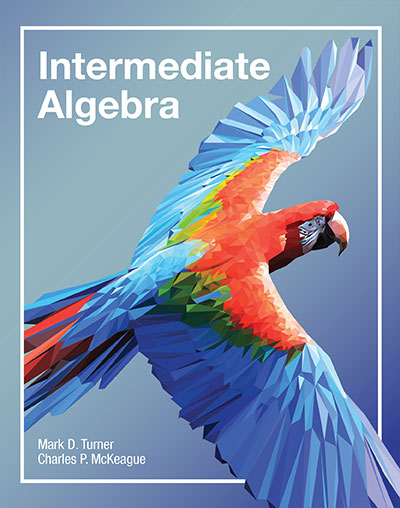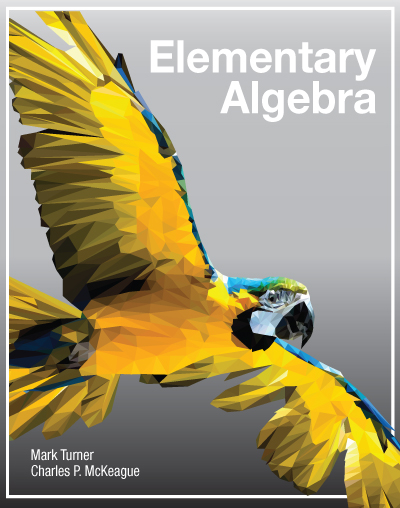# Introducing three new books from XYZ Textbooks

The new developmental math series by an award-winning, innovative teacher and one of the best-selling textbook authors of this generation.

### Elementary Algebra• Title: Elementary Algebra
• Author: Turner/McKeague
• Retail Price: eBook $45 /$98 print
• Wholesale Price: $75 print • ISBN: 978-1-63098-051-1 • Publication date: 10/1/15 • Elementary Algebra fits the traditional, one-semester, elementary algebra course. The prerequisite is Basic Math or Pre-algebra in college, or college prep math in high school. 1. Real Numbers and Algebraic Expressions 2. Linear Equations and Inequalities 3. Linear Equations and Inequalities in Two Variables 4. Systems of Linear Equations 5. Exponents and Polynomials 6. Factoring 7. Rational Expressions 8. Roots and Radicals 9. Quadratic Equations 1. REAL NUMBERS AND ALGEBRAIC EXPRESSIONS 1. Variables, Symbols, and the Order of Operations 2. The Real Numbers 3. Addition of Real Numbers 4. Subtraction of Real Numbers 5. Multiplication and Division of Real Numbers 6. Fractions 7. Properties of Real Numbers 2. LINEAR EQUATIONS AND INEQUALITIES 1. Simplifying Expressions 2. Addition Property of Equality 3. Multiplication Property of Equality 4. Solving Linear Equations 5. Formulas and Percents 6. Applications 7. More Applications 8. Linear Inequalities 3. LINEAR EQUATIONS AND INEQUALITIES IN TWO VARIABLES 1. Paired Data and Graphing Ordered Pairs 2. Graphing Linear Equations in Two Variables 3. More On Graphing: Intercepts 4. The Slope of the Line 5. Slope-Intercept Form 6. Point-Slope Form 7. Linear Inequalities in Two Variables 4. SYSTEMS OF LINEAR EQUATIONS 1. Solving Linear Systems by Graphing 2. The Elimination Method 3. The Substitution Method 4. Applications 5. EXPONENTS AND POLYNOMIALS 1. Multiplication with Exponents 2. Division with Exponents 3. Operations with Monomials 4. Addition and Subtraction of Polynomials 5. Multiplication with Polynomials 6. Binomial Squares and Other Special Products 7. Dividing a Polynomial by a Monomial 8. Dividing a Polynomial by a Polynomial 6. FACTORING 1. The Greatest Common Factor and Factoring by Grouping 2. Factoring Trinomials 3. More Trinomials to Factor 4. The Difference of Two Squares 5. The Sum and Difference of Two Cubes 6. Factoring: A General Review 7. Solving Equations by Factoring 8. Applications 7. RATIONAL EXPRESSIONS 1. Reducing Rational Expressions to Lowest Terms 2. Multiplication and Division of Rational Expressions 3. Addition and Subtraction of Rational Expressions 4. Equations Involving Rational Expressions 5. Applications 6. Complex Fractions 7. Proportions 8. Variation 8. ROOTS AND RADICALS 1. Definitions and Common Roots 2. Properties of Radicals 3. Simplified Form for Radicals 4. Addition and Subtraction of Radical Expressions 5. Multiplication and Division of Radical Expressions 6. Equations Involving Radicals 9. QUADRATIC EQUATIONS 1. More Quadratic Equations 2. Completing the Square 3. The Quadratic Formula 4. Complex Numbers 5. Complex Solutions to Quadratic Equations 6. Graphing Parabolas ### Intermediate Algebra• Title: Intermediate Algebra • Author: Turner/McKeague • Retail Price: eBook$45 / $98 print • Wholesale Price:$75 print
• ISBN: 978-1-63098-050-4
• Publication date: 10/1/15
•

Intermediate Algebra fits the traditional, one-semester, intermediate algebra course. The prerequisite is Elementary Algebra in college, or Algebra I in high school.

1. Real Numbers and Algebraic Expressions
2. Equations and Inequalities in One Variable
3. Linear Equations and Functions in Two Variables
4. Systems of Equations
5. Polynomials and Factoring
6. Rational Expressions and Rational Functions
7. Rational Exponents and Roots
9. Exponential and Logarithmic Functions
10. Sequences and Series
11. Conic Sections
1. REAL NUMBERS AND ALGEBRAIC EXPRESSIONS
1. The Real Numbers
2. Properties of Real Numbers
3. Arithmetic with Real Numbers
4. Exponents and Scientific Notation
2. EQUATIONS AND INEQUALITIES IN ONE VARIABLE
1. Linear Equations
2. Formulas
3. Applications
4. Linear Inequalities in One Variable and Interval Notation
5. Union, Intersection, and Compound Inequalities
6. Equations with Absolute Value
7. Inequalities Involving Absolute Value
3. LINEAR EQUATIONS AND FUNCTIONS IN TWO VARIABLES
1. The Rectangular Coordinate System and Graphing Lines
2. The Slope of a Line
3. The Equation of a Line
4. Linear Inequalities in Two Variables
5. Introduction to Functions
6. Evaluating Functions
7. Algebra and Composition with Functions
8. Variation
4. SYSTEMS OF EQUATIONS
1. Systems of Linear Equations in Two Variables
2. Systems of Linear Equations in Three Variables
3. Applications
4. Systems of LInear Inequalities
5. POLYNOMIALS AND FACTORING
1. Sums and Differences of Polynomials
2. Multiplication of Polynomials
3. Division of Polynomials
4. Greatest Common Factor and Factoring by Grouping
5. Factoring Trinomials
6. Factoring Special Products
7. Factoring: A General Review
8. Solving Equations by Factoring
6. RATIONAL EXPRESSIONS AND RATIONAL FUNCTIONS
1. Evaluating and Reducing Rational Expressions
2. Multiplication and Division of Rational Expressions
3. Addition and Subtraction of Rational Expressions
4. Complex Fractions
5. Equations with Rational Expressions
6. Applications
7. Rational Functions
7. RATIONAL EXPONENTS AND ROOTS
2. Rational Exponents
5. Multiplication and Division of Radical Expressions
7. Complex Numbers
1. The Square Root Property and Completing the Square
3. More on Solutions to Quadratic Equations
9. EXPONENTIAL AND LOGARITHMIC FUNCTIONS
1. Exponential Functions
2. The Inverse of a Function
3. Logarithms and Logarithmic Functions
4. Properties of Logarithms
5. Common Logarithms, Natural Logarithms, and Change of Base
6. Exponential and Logarithmic Equations
10. SEQUENCES AND SERIES
2. Series
3. Arithmetic Sequences
4. Geometric Sequences
5. The Binomial Expansion
11. CONIC SECTIONS
1. The Distance Formula and Circles
2. Parabolas
3. Ellipses and Hyperbolas
4. Second-Degree Inequalities and Nonlinear Systems

### Elementary and Intermediate Algebra• Title: Elementary and Intermediate Algebra
• Author: Turner/McKeague
• Retail Price: eBook $45 /$120 print

## Intermediate Algebra is available now!

Sign up to be notified as soon as the other books are published!